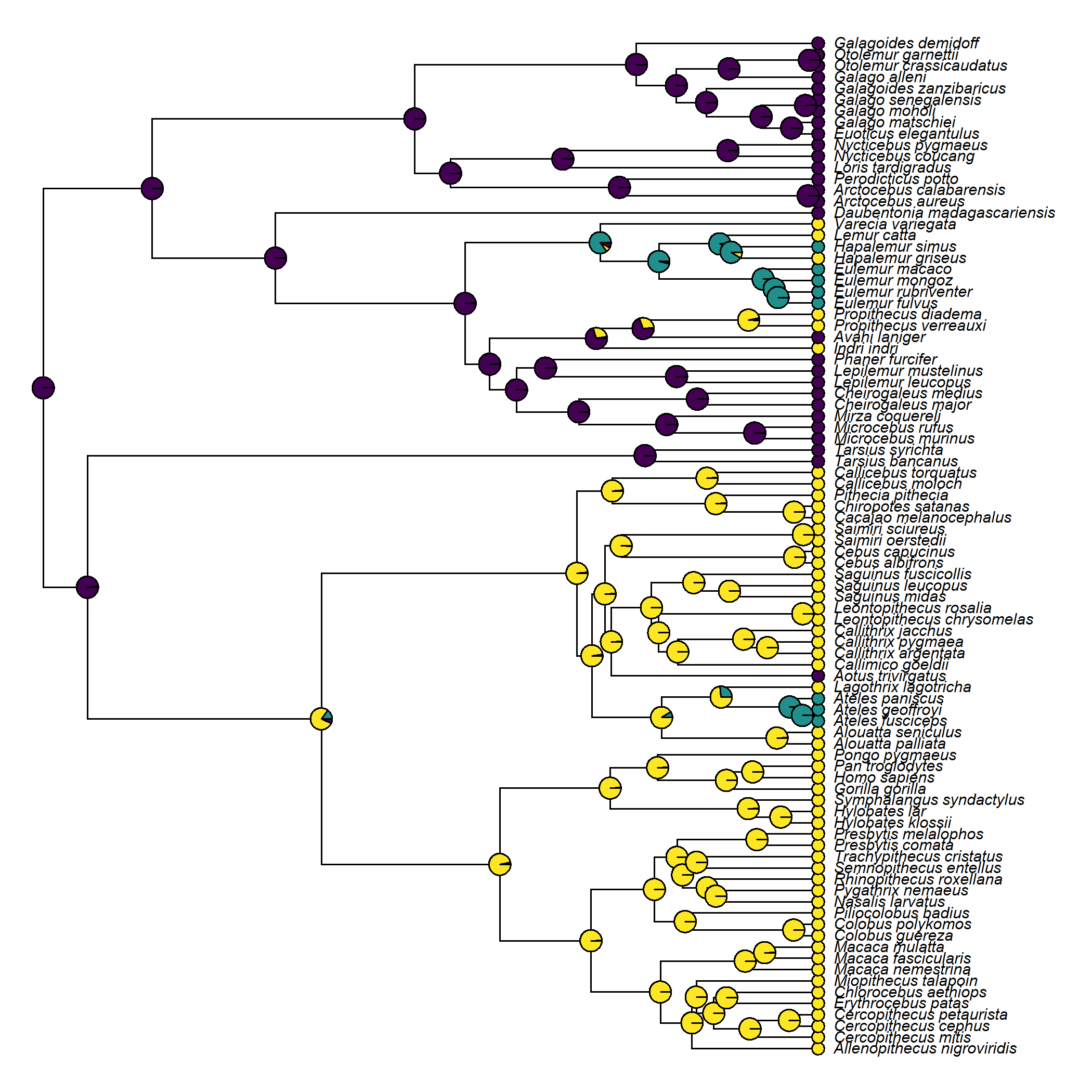## Tuesday, April 18, 2023

### Coloring the edges of a plotted tree by the marginal reconstruction with the highest likelihood

An R phylogenetics user asked the following:

“I would like to use the results of make.simmap to plot the edges of a tree by their most likely state. (Because my tree is really large and plotting pie charts at the nodes gets really polluted.)”

They had run into lots of problems, but this is not too difficult to do, so I thought I’d show a couple of solutions here.

One dilemma that we immediately face is that every node in the tree has a reconstructed state (rather than every edge), so which node should we use?

For simplicity, I thought it would make the most sense to simply graph the color of the most likely state in the descendant node or tip.

To illustrate it, I’ll use a simple example of diel activity pattern in primates with a phylogeny & dataset from Kirk & Kay (2004). Users wanting to follow along precisely will need to update phytools from GitHub.

``````library(phytools)
packageVersion("phytools")
``````
``````##  '1.7.6'
``````
``````data(primate.tree)
data(primate.data)
activity<-setNames(primate.data\$Activity_pattern,
rownames(primate.data))
``````

I’m going to start by doing simple marginal ancestral state reconstruction (rather than stochastic mapping, as in the query) using `phytools::ancr` as follows.

``````ard_model<-fitMk(primate.tree,activity,model="ARD",pi="fitzjohn",
rand_start=TRUE,smart_start=TRUE)
ard_model
``````
``````## Object of class "fitMk".
##
## Fitted (or set) value of Q:
##            Cathemeral   Diurnal Nocturnal
## Cathemeral  -0.050530  0.050530  0.000000
## Diurnal      0.002306 -0.004367  0.002061
## Nocturnal    0.002586  0.003565 -0.006151
##
## Fitted (or set) value of pi:
## Cathemeral    Diurnal  Nocturnal
##   0.003676   0.021704   0.974620
## due to treating the root prior as (a) nuisance.
##
## Log-likelihood: -29.714931
##
## Optimization method used was "nlminb"
``````
``````activity_anc<-ancr(ard_model)
activity_anc
``````
``````## Marginal ancestral state estimates:
##    Cathemeral  Diurnal Nocturnal
## 91   0.000014 0.000496  0.999490
## 92   0.005491 0.015299  0.979210
## 93   0.149800 0.782695  0.067505
## 94   0.032725 0.965831  0.001444
## 95   0.006652 0.993313  0.000036
## 96   0.001721 0.998274  0.000005
## ...
##
## Log-likelihood = -29.714931
``````
``````cols<-setNames(c("#21908CFF","#FDE725FF","#440154FF"),
sort(unique(activity)))
``````
``````plotTree(primate.tree,ftype="i",fsize=0.6,lwd=1,offset=0.5)
tiplabels(pie=ard_model\$data[primate.tree\$tip.label,],
piecol=cols,cex=0.3)
nodelabels(pie=activity_anc\$ace,piecol=cols,
cex=0.5)
``````Now, plotting each edge in the color with the highest marginal likelihood is easier than you probably think.

Just so we can be sure we got it right, I’m going to also overlay the marginal reconstruction pie charts on my nodes & tips.

Alright, here goes nothing!

``````allStates<-rbind(ard_model\$data[primate.tree\$tip.label,],
activity_anc\$ace)
edge.cols<-cols[apply(allStates,1,function(x)
which(x==max(x)))][primate.tree\$edge[,2]]
plot(primate.tree,edge.color=edge.cols,cex=0.6,no.margin=TRUE,
label.offset=2,edge.width=2,direction="upwards",
y.lim=c(0,95))
tiplabels(pie=ard_model\$data[primate.tree\$tip.label,],
piecol=cols,cex=0.3)
nodelabels(pie=activity_anc\$ace,piecol=cols,
cex=0.5)
``````